# ICSE Class 10 Physics 2022 Semester-1 Sample Paper

Question 1

(a) Choose the correct statement with respect to Refraction of light

1. The frequency always changes when light enters from one optical medium to another.
2. Absorption of light when it strikes the surface of a medium is refraction.
3. Speed of light changes when it enters from one optical medium to another of different optical density.
4. Speed of light does not change when it enters from one optical medium to another of different optical density.

(b) When a light ray enters from a denser medium to a rarer medium

1. The light ray bends towards the normal.
2. Angle of incidence is less than angle of refraction.
3. Speed of light decreases.
4. Speed of light remains unchanged.

(c) In the diagram shown below: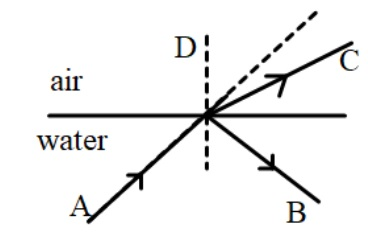1. B is incident ray and C is refracted ray.
2. A is incident ray and B is refracted ray.
3. C is incident ray and B is refracted ray.
4. A is incident ray and C is refracted ray.

(d) From the diagram shown below, identify the characteristics of the image that will be formed.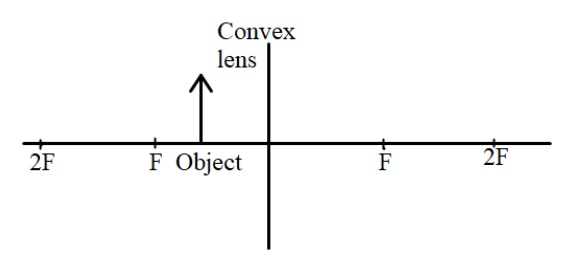1. Real.
2. Diminished.
3. Formed within the focal length.
4. Virtual.

(e) The wavelength of light in a medium A is 600 nm. The wave enters medium B of refractive index 1.5 with respect to medium A.

(i) Select the correct option from the following.

1. the wavelength of light becomes 1.5 times the initial wavelength.
2. the wavelength of light decreases.
3. the wavelength becomes half of initial wavelength.
4. the wave bends away from the normal.

(ii) The wavelength in medium B will be

1. 400 nm
2. 900 nm
3. 300 nm
4. Information is insufficient to calculate.

(f) The diagram below shows an image formed at a distance 36 cm from the lens LL’ of focal length 12 cm. With respect to this answer the questions that follow.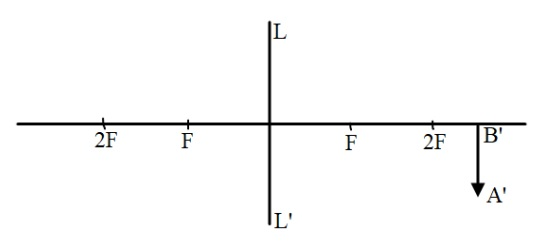(i) The position of the object on the left-hand side should be

1. between 12 cm to 30 cm from the lens.
2. beyond 24 cm from the lens.
3. between 12 cm to 24 cm from the lens.
4. within 12 cm from the lens.

(ii) Power of this lens is

1. - 8.33 D
2. + 8.4 D
3. + 8.33 D
4. - 8.4 D

(iii) The object distance with sign convention is

1. - 18 cm
2. - 15 cm
3. - 9 cm
4. + 18 cm

(iv) If the lens LL’ is replaced by another lens of same type but focal length 15 cm then for the same object distance

1. the size of the image decreases.
2. the size of the image increases.
3. the size of the image remains the same.
4. information is insufficient to conclude.

Question 2

(a) The usable form of mechanical energy is

1. Elastic potential energy
2. Kinetic energy
3. Gravitational potential energy
4. None of the given options

(b) One horsepower is equal to

1. 100 W
2. 735 W
3. 764 W
4. 746 W

(c) If A and B of the same mass can climb the third floor of the same building in 3 minutes and 5 minutes respectively, then the ratio of their powers of A is to B in an ideal situation is

1. 1:1
2. 3:5
3. The information is insufficient to form a conclusion.
4. 5:3

(d) If the centre of gravity of a metre scale of mass 80 g lies at the 45 cm mark, then which one of the following diagrams will show the balanced position of the scale.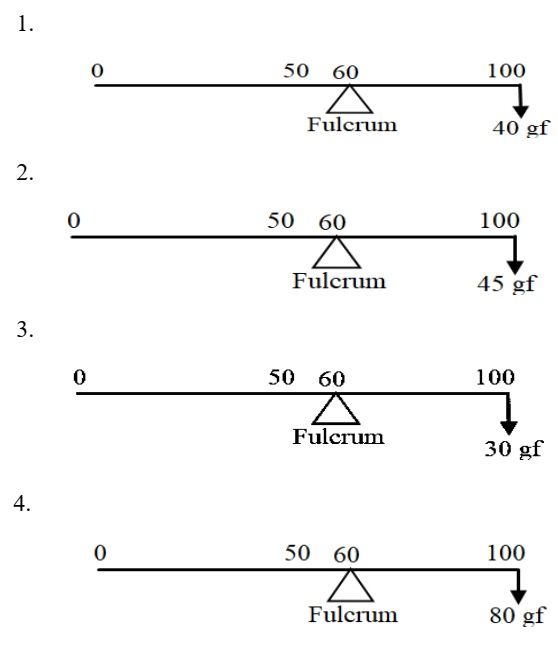(e) A body has kinetic energy 2500 J. If the mass of the body is 500 g.

(i) The velocity of the body is

1. √10 m/s
2. 10 m/s
3. 20 m/s
4. 100 m/s

(ii) The momentum of the body will be

1. 10 kg ms-1
2. 500√10 kg ms-1
3. 50 kg ms-1
4. 5 kg ms-1

(f) A girl at rest at gate of her society which is 3.2 m above the road comes down the slope AB on a cycle without paddling. [g = 10 N/kg]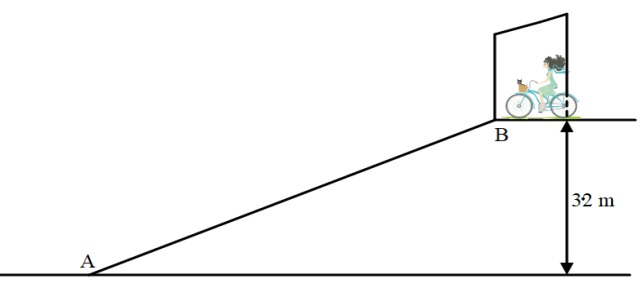(i) The mechanical energy possessed by the girl at B is

1. Vibrational kinetic energy.
2. Translational kinetic energy
3. Elastic potential energy.
4. Gravitational potential energy.

(ii) The velocity with which girl reaches point A is

1. 32 m/s
2. 10 m/s
3. 8 m/s
4. Insufficient information to calculate velocity.

(iii) If the mass of the girl is 40 kg then the kinetic energy of the girl at A is [Assuming no loss of energy.]

1. 1280 J
2. 1600 J
3. 400 J
4. 3200 J

(iv) The potential energy of the girl (of mass 40 kg) when she reaches the midpoint of the slope of AB

1. 800 J
2. 200 J
3. 1600 J
4. 640 J

Question 3

(a) Mechanical advantage (M.A.), load (L), and effort (E) are related as

1. M.A. = L x E
2. M.A. = E/L
3. M.A. x E = L
4. M.A. x L = E

(b) Which one of the following statements is correct?

1. A machine is used to have more output energy as compared to input energy.
2. Mechanical advantage of a machine can never be greater than 1.
3. If a machine gives convenience of direction, then its mechanical advantage should be greater than 1.
4. For a given design of a machine, even if the mechanical advantage increases, the velocity ratio remains the same.

(c) If a block and tackle system with convenient direction has 3 movable pulleys, then its velocity ratio

1. is either 6 or 7
2. should be 6
3. should be 7
4. is 3

(d) Work done by a body moving on a circular track is zero at every instant because

1. displacement is zero.
2. displacement is perpendicular to the centripetal force.
3. there is no force acting.
4. reason is not mentioned in the other options.

(e) Identify the conditions required to hear a clear and distinct echo by humans, in air

1. The reflecting surface should be rough.
2. The size of the reflecting surface should be smaller than the wavelength of sound.
3. Sound should not be reflected back within 0.1 s.
4. The incident sound should have frequency more than 25000 Hz.
5. The size of the reflecting surface should be larger than the wavelength of sound.

(f) A person standing in front of a vertical cliff fires a gun and hears its echo in 3s. The speed of sound in air is 340 m/s.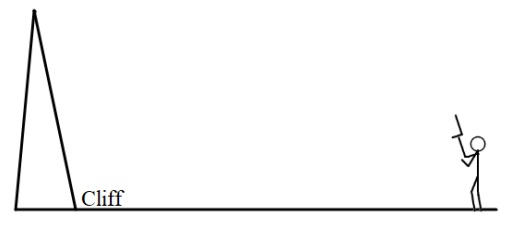(i) The distance at which the person is standing in front of the cliff is

1. 1020 m
2. 510 m
3. 340 m
4. 680 m

(ii) If the person wants to hear the echo 0.5 s earlier, then how much distance should he move, toward or away from the cliff?

1. 595 m away from the cliff
2. 255 m towards the cliff
3. 85 m towards the cliff
4. 255 m away from the cliff

(iii) Another person stands behind this person, in the same line with him and the cliff, at a distance of 170 m and fires a gun in the air. What are the consecutive intervals of time at which the first person hears two sounds?

1. 0.5 s and 3 s
2. 1 s and 3 s
3. 1 s and 4 s
4. 0.5 s and 3.5 s

(iv) If the speed of sound changes to 350 m/s then how much distance should the person move towards or away from the cliff, in order to hear the echo in the same time? (i.e. in 3 s)?

1. 25 m away
2. 7.5 m away
3. 20 m away
4. 15 m away

Question 4

(a) Assuming all lenses shown below are of the same material, state which lens has the maximum power.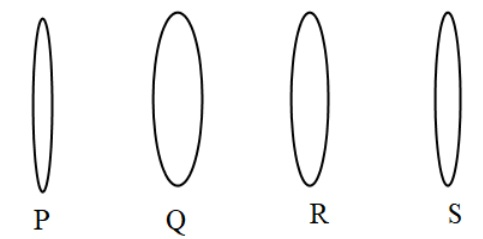1. R
2. P
3. Q
4. S

(b) In an electric cell while in use, the change in energy is from:

1. Chemical to mechanical
2. Chemical to electrical
3. Electrical to mechanical
4. Electrical to chemical

(c) The diagram below shows a pendulum having a bob of mass 80 g. A and C are extreme positions and B is the mean position. The bob has velocity 5 m/s at position B. [g = 10N/kg]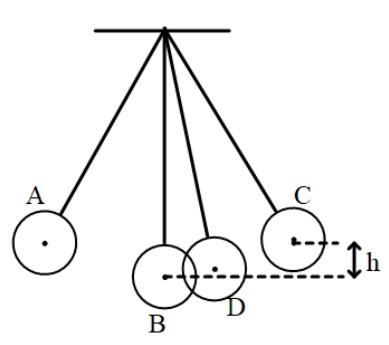(i) Which one of the following statements is correct?

1. At point A the bob has only kinetic energy.
2. At point B the bob will have only potential energy.
3. At point B the bob will have maximum kinetic energy.
4. At point D the bob will have more potential and less kinetic energy.

(ii) The height h is

1. 1.25 cm
2. 125 m
3. 1.25 m
4. 0.125 m

(d) (i) Select correct options for Total internal reflection in a medium.

1. Can take place in an optically denser medium as compared to an optically rarer medium.
2. Takes place for any angle of incidence greater than 42 degree.
3. This reflection does not obey the laws of reflection.
4. Can take place if the angle of incidence in a denser medium is less than the critical angle.

(ii) Diamonds glitter in the dark because

1. They emit light.
2. They have a very small critical angle due to very high refractive index.
3. Due to the fluorescence.
4. Chemical reaction in the diamond produces light energy.

(e) The diagram shows the path of light through a right-angled prism of critical angle 42°.

Observe the diagram and answer the questions that follow.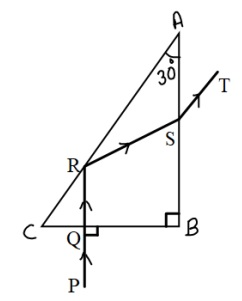(i) The phenomenon at the surface AC is

1. Refraction
2. Partial reflection
3. Total internal reflection
4. Scattering

(ii) The angle of incidence at the surface AC is

1. 30°
2. 45°
3. 60°
4. 90°

(iii) The angle of incidence at the surface AB is

1. 30°
2. 45°
3. 60°
4. 90°

(iv) Which of the following statement is wrong?

1. Speed of light ray PQ is equal to the speed of light ray ST.
2. Speed of light ray QR is equal to the speed of light ray RS.
3. Speed of light ray PQ is greater than the speed of light ray RS.
4. Speed of light ray RQ is greater than the speed of light ray ST.